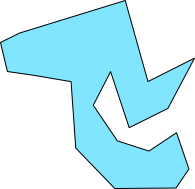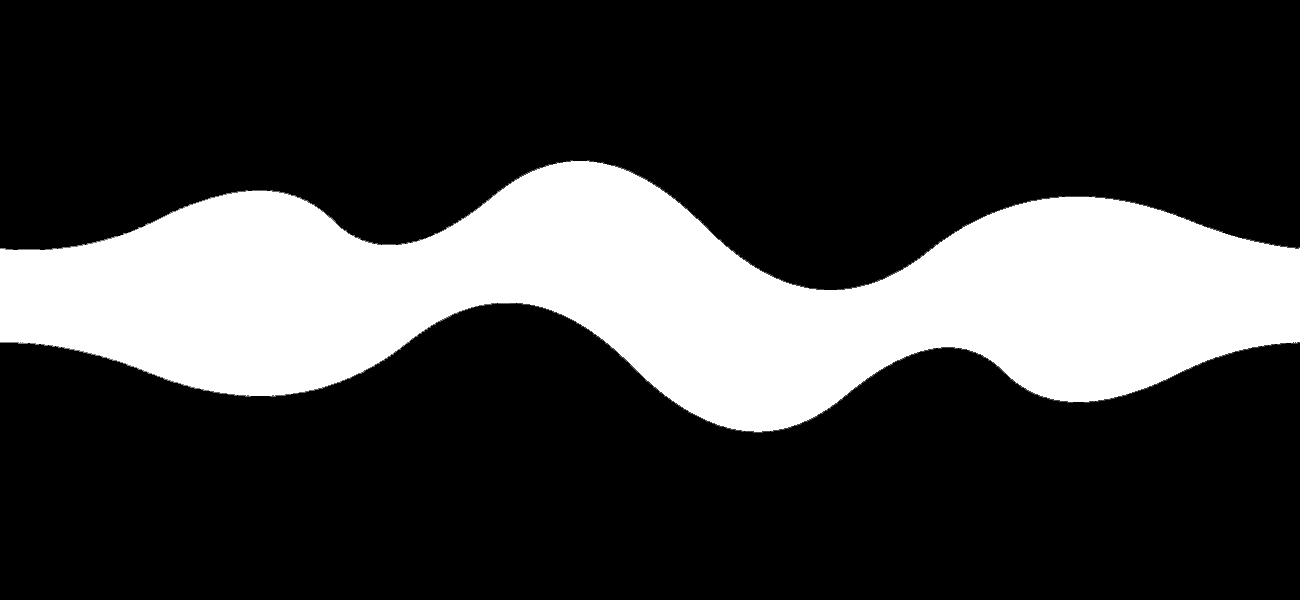# Simple Collision Detection

Rectangular Collision

This simple example demonstrates collisions detection between two rectangular objects. Note that this method will only work when both rectangles are axis-aligned, and only with rectangles!

However, it's possible to use this approach with invisible bounding boxes around more complex shapes. In many cases, as in old arcade games, the user may not even notice the collisions are based purely on rectangles.

```int rctl1X; // rectangle One x position
int rctl1Y; // rectangle One y position
int rctl1W = 40; // rectangle One Width
int rctl1H = 70; // rectangle One Height
//
int rctl2X; // rectangle One x position
int rctl2Y; // rectangle One y position
int rctl2W = 100; // rectangle 2 Width
int rctl2H = 100; // rectangle 2 Height

void setup() {
size(800, 600);
noStroke();
rectMode(CENTER);
rctl2X = width/2;
rctl2Y = height/2;
}

void draw() {
background(255);
rctl1X = mouseX;
rctl1Y = mouseY;
boolean collision = checkCollision(rctl1X, rctl1Y, rctl1W, rctl1H, rctl2X, rctl2Y, rctl2W, rctl2H);
if (collision) {
fill(70, 0, 0);
} else {
fill(255, 0, 0);
}
rect(rctl1X, rctl1Y, rctl1W, rctl1H);
rect(rctl2X, rctl2Y, rctl2W, rctl2H);
}

boolean checkCollision(int r1x, int r1y, int r1w, int r1h, int r2x, int r2y, int r2w, int r2h) {
// store the locations of each rectangles outer borders
int top1 = r1y-r1h/2;
int bottom1 = r1y+r1h/2;
int right1 = r1x+r1w/2;
int left1 = r1x-r1w/2;
int top2 = r2y-r2h/2;
int bottom2 = r2y+r2h/2;
int right2 = r2x+r2w/2;
int left2 = r2x-r2w/2;

if (top1>bottom2 || bottom1<top2 || right1<left2 || left1>right2) {
return false;
} else {
return true;
}
}

```

Circular Collision

The following example demonstrates a simple method for checking collisions between round objects. As in the above example, it can be used to create invisible bounding boxes for more complex shapes.

```ArrayList<Ball> ballList = new ArrayList<Ball>();
Ball mouseBall;
void setup()
{
size(600, 800);
mouseBall = new Ball(mouseX, mouseY, 20);
}
void draw()
{
background(255);
mouseBall.update(mouseX, mouseY);

for (int i = 0; i<ballList.size(); i++) {
ballList.get(i).drawBall();
}
mouseBall.drawBall();
}
class Ball {
PVector _position = new PVector();
int colour = 250;
public Ball(int x, int y, int r) {
_position.set(x, y, 0);
}
void update(int x, int y) {
_position.set(x, y, 0);
}

void drawBall() {
pushMatrix();
fill(colour, 0, 0);
noStroke();
translate(_position.x, _position.y);
popMatrix();
}

void checkCollision(PVector position, int radius) {
float distance = PVector.dist(_position, position);
println("hit");
colour = 60;
} else {
colour = 255;
}
}
}

```

Point and Polygon

Sometimes it is only necessary to check if one specific points is inside a very complex shape. This complex shape can even be convex, which means that the edges of the polygon do not only point to the outside, but maybe also to the inside.But there is a simple solution to check, if a point is located inside or outside this complex shape. W. Randolph Franklin created this method to check, if a point is located inside an Array of points. This is an adapted version for processing.

PNPoly
```boolean pnpoly(PVector[] vertices, float testx, float testy)
{
int i, j;
boolean c = false;
for (i = 0, j = vertices.length-1; i < vertices.length; j = i++) {
if ( ((vertices[i].y>testy) != (vertices[j].y>testy))
&& (testx < (vertices[j].x-vertices[i].x) * (testy-vertices[i].y) / (vertices[j].y-vertices[i].y) + vertices[i].x) )
c = !c;
}
return c;
}```

Bitmap Collision

For complex shapes, it's possible to create a reference bitmap to indicate hit areas. This reference can be black and white images, which correlates exactly to an image or shape that will be displayed on the screen. This method works well for game maps, and in situations where you are testing a simple shape against a complex one.

Out background image:Our collision reference: